Courses

# MCQ (Previous Year Questions) - Hydrocarbons (Level 2)

## 25 Questions MCQ Test Chemistry for JEE Advanced | MCQ (Previous Year Questions) - Hydrocarbons (Level 2)

Description
This mock test of MCQ (Previous Year Questions) - Hydrocarbons (Level 2) for JEE helps you for every JEE entrance exam. This contains 25 Multiple Choice Questions for JEE MCQ (Previous Year Questions) - Hydrocarbons (Level 2) (mcq) to study with solutions a complete question bank. The solved questions answers in this MCQ (Previous Year Questions) - Hydrocarbons (Level 2) quiz give you a good mix of easy questions and tough questions. JEE students definitely take this MCQ (Previous Year Questions) - Hydrocarbons (Level 2) exercise for a better result in the exam. You can find other MCQ (Previous Year Questions) - Hydrocarbons (Level 2) extra questions, long questions & short questions for JEE on EduRev as well by searching above.
QUESTION: 1

Solution:
QUESTION: 2

Solution:
QUESTION: 3

### Statement-1 : 1-butene on reaction with HBr in the presence of a peroxide produces 1-bromobutane. Statement-2 : It involves the formation of a primary radical.               [IIT 2000]

Solution:
QUESTION: 4

In the presence of peroxide, hydrogen chloride and hydrogen iodide do not give anti–Markonikoff addition to alkene because –                            [IIT ‘2001]

Solution:
QUESTION: 5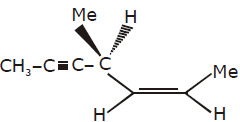Hydrogenation of the above compound in the presence of poisoned paladium catalyst gives –           [IIT ‘2001]

Solution:
QUESTION: 6

The reaction of propene with HOCl proceeds via the addition of –             [IIT ‘2001]

Solution: Hi adit, basicly HOCl reaction is 2 step reaction. Step 1 - in step one , first HOCL decomposes into OH - & CL+. And 'CL - ' attacks on corner hydrogen of propane. & remove 'H+' Step 2- Now, this H+ react with OH- and forms Water. So in first step CL + is formed .
QUESTION: 7

Statement-1 : Addition of bromine to trans-2-butene yields meso-2, 3-dibromo butane.

Statement-2 : Bromine addition to an alkene is an electrophilic addition.           [IIT 2001]

Solution:
QUESTION: 8

Consider the following reactions –                 [IIT ‘2002]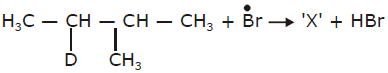Identify the structure of the major product ‘X’

Solution:
QUESTION: 9

Identify a reagent from the following list which can easily distinguish between 1–butyne and 2-butyne-

[IIT ‘2002]

Solution:
QUESTION: 10

Identify the set of reagents/reaction conditions 'X' and 'Y' in the following set of transformation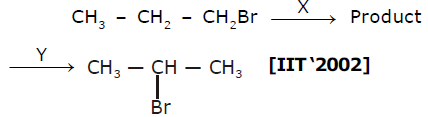Solution:
QUESTION: 11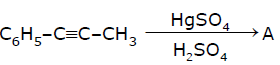[IIT 2003]

Solution:
QUESTION: 12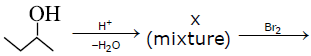5 compounds of molecular formula C4H8Br2 Number of compounds in X will be:            [IIT ‘2003]

Solution:
QUESTION: 13

2–hexyne can be converted into trans–2–hexene by the action of :             [IIT ‘2004]

Solution:
QUESTION: 14

2-phenyl propene on acidic hydration, gives                 [IIT ‘2004]

Solution:
QUESTION: 15

How many chiral compounds are possible on mono chlorination of 2-methyl butane ?          [IIT ‘2004]

Solution:
QUESTION: 16

1–bromo–3–chlorocyclobutane when treated with two equivalents of Na, in the presence of ether which of the following will be formed?                   [IIT ‘2005]

Solution:
QUESTION: 17

Cyclohexene is best prepared from cyclohexanol by which of the following          [IIT ‘2005]

Solution:
QUESTION: 18

CH3–CH=CH2 + NOCl → P Identify the adduct.                    [IIT 2006]

Solution:
QUESTION: 19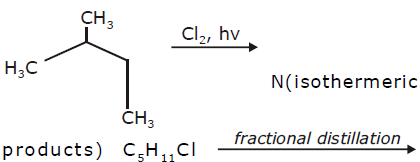M(isomeric products). What are N and M ?                   [IIT ‘2006]

Solution:
QUESTION: 20

The number of structural isomers for C6H14 is                          [IIT 2007]

Solution:
QUESTION: 21

The number of stereoisomers obtained by bromination of trans-2-butene is                 [IIT 2007]

Solution:
QUESTION: 22

The reagent(s) for the following conversion,                            [IIT 2007]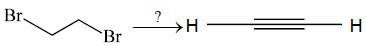is / are

Solution:
QUESTION: 23

The correct stability order for the following species is                     [IIT ‘2008]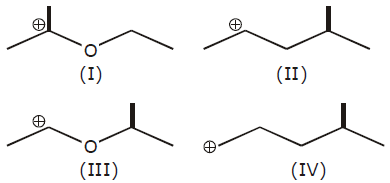Solution:
QUESTION: 24

In the following carbocation, H/ CH3 that is most likely to migrate to the positively charged carbon is

[IIT 2009]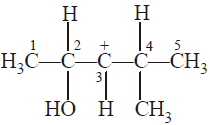Solution:
QUESTION: 25

Intermediate produced when propene react with HCl in presence of peroxide      [IIT 2009]

Solution: